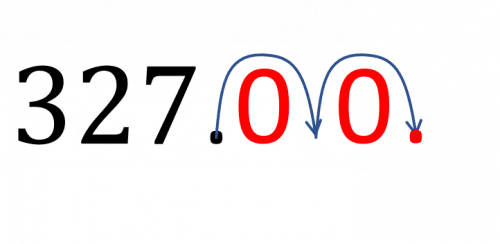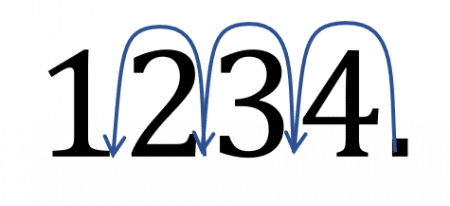# Multiplying and dividing by 10, 100, 1000

KS3Level 1-3AQAEdexcelOCRWJEC

## Multiplying and dividing by 10, 100, 1000

When we multiple by 10, 100 or 1000 we move the decimal place to the right. When we divide by 10, 100 or 1000 we move the decimal place to the left.

Make sure you are happy with the following topics before continuing.

## Skill 1: Multiplying by 10, 100, 1000

When multiplying by $10, 100, 1000$ move the decimal place to the right, the same number of places as zeros in the number.

Multiplying by $10$ – decimal place $1$ space to the right

Multiplying by $100$ – decimal place $2$ space to the right

Multiplying by $1000$ – decimal place $3$ space to the right

Example: Calculate $327 \times 100$

Here we need to more the decimal place 2 spaces to the right, writing zeros in any new places created.After moving the decimal place 2 spaces to the right and add the zeros in the spaces created, this gives the answers $32700$

$327 \times 100 = 32700$

Level 1-3KS3AQAEdexcelOCRWJEC

## Skill 2: Dividing by 10, 100, 1000

When Dividing by $10, 100, 1000$ move the decimal place to the left, the same number of places as zeros in the number.

Multiplying by $10$ – decimal place $1$ space to the left

Multiplying by $100$ – decimal place $2$ space to the left

Multiplying by $1000$ – decimal place $3$ space to the left

Example: Calculate $1234 \div 1000$

Here we need to more the decimal place 3 spaces to the left.The decimal place is moved three places to the left.

This gives the answer to be $1.234$

$1234 \div 1000 = 1.234$

Level 1-3KS3AQAEdexcelOCRWJEC

## Multiplying and dividing by 10, 100, 1000 Example Questions

Here we are multiplying by $100$ this will mean we move the decimal place $2$ places to the right.

$17\times100 = 1700$Gold Standard Education

Here we are multiplying by $1000$ so we must move the decimal place $3$ places to the right.

$219.65\times1000= 219650$Gold Standard Education

As we are dividing by $100$ we must move the decimal place $2$ places to the left.

$3101 \div 100 = 31.01$Gold Standard Education

As we are dividing by $1000$ we must move the decimal place $3$ places to the left.

$12\div1000= 0.012$Gold Standard EducationGold Standard Education

Level 1-3GCSE

Level 1-3GCSE